# Mathematical Modeling of Multilayered Skin with Embedded Tumor Through Combining Laser Ablation and Nanoparticles: Effects of Laser Beam Area, Wavelength, Intensity, Tumor Absorption Coefficient and Its Position

Mathematical Modeling of Multilayered Skin with Embedded Tumor Through Combining Laser Ablation and Nanoparticles: Effects of Laser Beam Area, Wavelength, Intensity, Tumor Absorption Coefficient and Its Position
Faculty of Medicine and Public Health, HRH Princess Chulabhorn College of Medical Science, Chulabhorn Royal Academy, Bangkok 10210, Thailand

Center of Excellence in Electromagnetic Energy Utilization in Engineering (C.E.E.E.), Department of Mechanical Engineering, Faculty of Engineering, Thammasat University (Rangsit Campus), Pathumthani 12120, Thailand

Corresponding Author Email:
Page:
89-100
|
DOI:
https://doi.org/10.18280/ijht.390109
14 August 2019
|
Accepted:
6 September 2020
|
Published:
28 February 2021
| Citation

OPEN ACCESS

Abstract:

Laser ablation is gaining acceptance as a cancer treatment technique because of its localized energy delivery to the tumorous tissue. The combination of laser ablation with nanoparticles allows for more precise targeting of the ablative area with less damage to adjacent healthy tissue. Nevertheless, heat damage to adjacent tissue is still a potential concern. Therefore, mathematical modeling of laser-tissue interactions is a necessary part of clinical treatment planning. In this study, the temperature distribution during laser-induced thermotherapy in cancer treatment investigates using a multilayered skin (epidermis, dermis, subcutaneous fat, and muscle) with an embedded tumor model. The effects of related parameters systematically investigate; wavelength, laser intensity, beam area, tumor absorption coefficient, tumor position, tumor blood perfusion rate, and irradiation time. Mathematical modeling in this study is solved by finite element method (FEM) via COMSOLTM Multiphysics Software. Laser absorption and thermal phenomena are described by Beer-Lambert’s law and Pennes’s bioheat equation.

Keywords:

laser ablation, bioheat transfer, tumor, cancer, skin, numerical simulation

1. Introduction

Skin cancer is a growing public health problem because the incidence of skin cancer has been increasing over recent decades. The major determinant of skin cancers development causes by ultraviolet radiation. It incurs dna damage and genetic mutations  as the results of abnormally and uncontrollably cells growth. These cluster of cells may form a mass and called tumor. It can be developed to cancerous tumor and spread to adjacent tissues as well as other cells which located far away .

There are many alternative techniques for cancer treatment such as ablation methods from laser, radiofrequency, microwave, high frequency focused ultrasound and cryosurgery and so on. All of these techniques could destroy cancer cells while sparing adjacent healthy tissue as well as delivery in minimally invasive, allowing less pain and providing shorter recovery time. Among the mentioned techniques, laser ablation shows attractive possibility of being effective way due to its precise and deliver energy directed into focused target tissue. As well as potential of combining of laser ablation with nanoparticles, ablative area could be selectively . Even though laser ablation treatments effectively kill cancerous tumors with its potential. Undesired thermal energy could be happened if the power is not adjusted appropriately. Therefore, effectively preplanning before actual operation is necessary.

In the past, thermal modeling of the ablation process in human tissue is mostly based on Pennes’s bioheat equation . Because of its simplification, the bioheat transfer model has been employed as the governing equation to analyzed the transient temperature distribution of the tumor during laser-induced thermotherapy by various researchers over the past decade.

Sazgarnia et al.  analyzed heat transfer and blood perfusion of tumor with surrounding tissue for tumor photothermal therapy by using finite element method (FEM). Pennes’s bioheat equation was implied and thermal effects of local blood perfusion were studied. Bhowmik et al.  represented mathematical modeling of laser and high-frequency focused ultrasound for breast tumors ablation. The thermal phenomena as well as induced thermal damage in breast tumors are evaluated by conducting of bioheat equation, couple with thermal damage model. The effects of heat transfer on tumor volume and irradiation time were investigated.

Luna et al.  investigated mathematical model of skin tumor by boundary element method with the simulated annealing technique. The temperature profiles with different sizes of tumors are described. Pennes's bioheat equation with specific thermal properties value of skin and tumors was analyzed in this study. The thermal analysis in tumors during laser photothermal therapy, induced by gold nanorods is represented by Manuchehrabadi et al. . The laser energy absorption in the tumor was simulated by FEM and the effects of absorption and scattering coefficients of tumors on the generated heating pattern were examined.

Fasano et al.  studied a numerical modeling of laser-induced thermotherapy for cancer treatment. The radiation transport equation coupled with a bioheat equation was used to approximate heat diffusion and the coagulated zone. The effect of coolant flow was analyzed. In addition, comparisons between experiments and simulations have been performed. Marqa et al.  carried out the numerical simulation on temperature distribution and thermal damage of focal laser ablation in prostate cancer model. The FEM is used to solve the bioheat and thermal damage equations.

Ma et al.  developed a two-layer tissue model. The thermal distributions during laser-induced interstitial thermo-therapy (LITT) were predicted by analysis of Pennes’s bioheat equation. Deng and Liu  solved the transient bioheat transfer problem with non-linear boundary conditions on three-dimensional skin embedded with tumor model. The temperature distribution as well as blood perfusion in the tumor are investigated. The effects of tumor size and number on the sensitivity of thermography were studied, and three layers of the skin model were represented.

In addition, the theoretical of Beer-Lambert’s law that was represented by Strong , has widely and generally used on the research work. To illustrate, Pual et al.  studied about the cooling effects of large blood vessels inside tissues during laser irradiation. A volumetric heat source term for laser heating is based on Beer–Lambert’s law and was calculated by bioheat equation. In the same way, Fanjul-Vélez et al.  analyzed temperature distribution in laser irradiated biological tissues based on a 3D multilayered model. Beer–Lambert’s law coupled with the bioheat equation were used for analysis as well.

However, most previous studies have mainly focused on modeling and the influence of specific parameters. Some publications performed systematic studies of the effects of operating parameters such as laser wavelength, laser irradiation intensity, tumor position, tumor absorption coefficient, laser irradiation beam area and irradiation time on heat transfer in the layered skin with an embedded tumor, although it directly affects the therapeutic heat transfer effect during cancer treatment by the laser. In practical ways, these effects lead to an enhanced heat transfer process and thermal absorption inside target tissue. This can cause change in temperature in skin tumor model. Therefore, to provide adequate information on the appropriate level of laser transition from the laser instrument, it is essential to consider all of the previously mentioned analyzed parameters.

In this study, the temperature distribution during laser-induced thermotherapy in cancer treatment is investigated with a developed multilayered skin (epidermis, dermis, subcutaneous fat and muscle) with an embedded tumor model. The effects of wavelength, intensity, beam area, tumor absorption coefficient, tumor position, tumor blood perfusion rate and irradiation time will be systematically investigated. The FEM is used for numerical simulation in this study. The spatial transient temperature distribution is analyzed by Pennes’s bioheat model and laser energy absorption is described following Beer-Lambert's law.

Mathematical modeling in this study can be usefully technique in predicting the result and representation of physical phenomena that would be happened in advance. In order to achieve an optimum outcome for cancerous tumor treatment by laser ablation, and also to optimize the effective parameters to get the optimal laser dosage, these related parameters should be appropriately and carefully adjusted to prevent the damage of healthy tissue. The obtained results from this study would provide more understanding in complicated medical process and could be the guideline for efficiency treatment planning before actual operation. By the way, even this model has been developed by applying more complexity. Carrying on more advanced study still be needed in order to mimic to get closer to realistic situation.

2. Formulation of the Problem

In the laser-induced thermotherapy process of multilayered skin tissue with an embedded tumor (Figure 1), the denaturation process in this tissue is essentially due to the heating effect, to obtain a large temperature increase in the tumor while limiting the normal tissue temperature.

## 1.png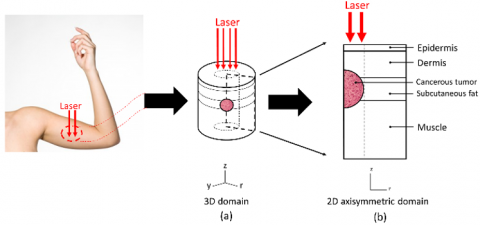Figure 1. Human multilayered skin with embedded tumor model, (a) 3D human skin with embedded tumor model with laser irradiation, (b) 2D human skin with embedded tumor model with laser irradiation

In this study, the values of tumor absorption coefficients (a) are considered as 4, 10 and 15 m-1. These values are referred to the energy adsorbent substance that used to inject into the tumor, namely nanoparticles. This substance can act as different intense light absorbers that leads to increasing of the temperature inside the embedded tumors, owing to the laser energy absorbed. This provides the potential ability of thermal delivery from laser-induced thermotherapy, the thermal energy is focused and tumor or tissue target would be destroyed directly.

However, in the beginning, the experimental laser treatment could not be conducted in vivo (studies are conducted in animals including humans and whole plants) because of ethical considerations. The numerical simulations are carried out instead. The analysis of heat transfer within multilayered skin with embedded tumor is presented in Section 3. The governing equations as well as its boundary conditions are numerically solved by using the FEM via COMSOLTM Multiphysics Software.

3. Methods and Model

A numerical model has been formulated to predict the thermal phenomena within the skin and embedded tumor during the laser-induced thermotherapy process. In order to different layer of skin provides different thermal and optical property values which result in change of thermal phenomena, such as value of tissue density, specific heat, thermal conductivity, metabolic heat generation and absorption coefficient. It can explain that the multilayered skin tissue structure can affect to heat transfer process. Thus, the mathematical model of skin tissue in this study, is developed to be multilayer and the details of it are describe in section 3.1. The first step in evaluating the effects of a certain exposure of the multilayered skin to laser irradiation is to determine the energy transfer process and its spatial distribution. Then, energy absorption which results in change in temperature inside tissue as well as other processes of transport phenomena can be considered.

3.1 Physical model

Figure 2 presents the developed skin with embedded tumor model used in this study. Although human skin is a complex heterogeneous tissue, a 2D skin model was constructed in an axis symmetric plane with a cross section of four different layers, epidermis, dermis, subcutaneous fat and muscle (viewed from the surface), and an embedded tumor in the cylindrical coordinate system, as demonstrated in Figure 2(b). Here, the two-dimensional view of the healthy tissue and embedded tumor that is located exactly in the center of a larger plane is performed. In this study, the embedded tumor located at depths of 3 mm and 8 mm from the surface of the skin were selected.

The laser spot in this study is assumed to be circular and irradiate perpendicularly on skin, where the heat source was confined. To simulate thermal behavior during laser-induced thermotherapy of this embedded tumor, we consider a cross section on this embedded tumor that is assumed to be circular in shape, with a diameter of 4 mm. Besides, multilayered skin tissue is approximately assumed to be isotropic and homogeneous medium in the same layer, indicating that there is no difference in their thermal and optical parameters in the same layer, as summarized in Table 1.

## 2.png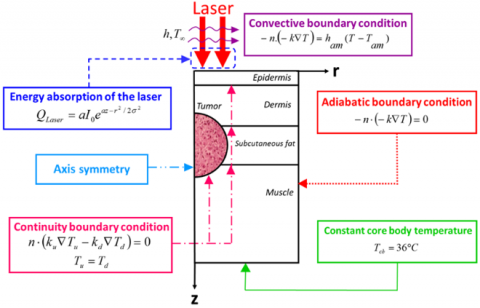Figure 2. Boundary conditions and physical domain

Table 1. Thermal properties and optical properties of tissues [16-20]

 Epidermis Dermis Subcutaneous fat Muscle Tumor Thickness (mm) 0.05 1.95 2.0 8.0 4.0 (Diameter) Tissue density, $\rho$ (kg. m-3) 1200.0 1090.0 1210.0 1085.0 1030.0 Specific heat of tissue, C ( J .kg-1. K-1) 3950.0 3350.0 2240.0 3800.0 3852.0 Thermal conductivity, k (W. m-1. K-1) 0.24 0.42 0.194 0.51 0.558 Blood perfusion, $\omega_{b}$ (1. s-1) 0.0 0.0031 0.0031 0.0027 0.0063 Metabolic heat generation, Qmet (W. m-3) 368.0 368.0 368.0 684.2 3680.0 Absorption coefficient, a (m-1), 532 nm 9.0 0.24 0.24 1.0 4.0, 10.0, 15.0 Absorption coefficient, a (m-1), 800 nm 5.3 0.24 0.24 1.0 4.0, 10.0, 15.0 Scattering coefficient, b (m-1) 5.0 The radius of laser beam, $\sigma$ (mm) 1.0, 2.0 Tumor depth position (mm) 3.0, 8.0 Ambient temperature, Tam (℃) 25.0 Initial temperature, T0 (℃) 36.0 Blood temperature, Tb (℃) 36.0 Convection heat transfer coefficient, h (W. m-2. K-1) 10.0 Blood density, $\rho_{b}$ (kg. m-3) 1060.0 Specific heat of blood, Cb (J .kg-1. K-1) 3660.0 Laser irradiation intensity, I (W. mm-2) 1.0, 2.0

3.2 Equations for heat transfer analysis

To simulate the laser-skin interaction process, a model of the unsteady heat transfer, as well as the boundary conditions, is investigated. Heat transfer analysis within the multilayered skin and embedded tumor during the laser-induced thermotherapy process is modeled in a 2D domain that is constructed in an axisymmetric plane with four different layers and an embedded tumor following Figure 1(b). In order to the problem simplification, the assumptions are presumed as following:

1. The substance in the tissue has no phase change.

2. The thermal and optical properties is assumed to be constant in the same layer.

3. There is no chemical reaction appear in the tissue.

4. The laser deposition term within tissue is described by Beer-Lambert's law.

5. The contact surfaces between each tissue are assumed to be smooth condition.

6. Unsteady heat transfer is considered.

7. The effect of mechanical deformation is negligible.

8. All types of tissue in this model are assumed to be homogeneous and isotropic.

9. Model is assumed to be two dimensional axial symmetrical model.

The temperature distribution within the human skin in this study, is evaluated by Pennes’ bioheat equation. The thermal phenomena varying time can be calculated by transient bioheat equation and can be written as:

$\rho C \frac{\partial T}{\partial t}=\nabla \cdot(k \nabla T)+\rho_{b} C_{b} \omega_{b}\left(T_{b}-T\right)+Q_{m e t}$$+Q_{\text {Laser }}$     (1)

where, r is density (kg.m-3), C is the heat capacity (J.kg-1.K-1), T is temperature (℃), k is the thermal conductivity of the tissue (W. m-1. K-1), w is blood perfusion rate (s-1), Q is heat generation (W. m-3) and subscript b is defined as value of blood, met is metabolic heat source and laser is external heat source from laser irradiation.

3.3 Boundary condition

According to the axisymmetric plane, as shown in Figure 1b, the boundary conditions and the physical domain are indicated in Figure 2. The upper surface of human multilayered skin (z=0) is considered to have a convective boundary condition:

$-n \cdot(-k \nabla T)=h_{a m}\left(T-T_{a m}\right)$     (2)

where, Tamis the ambient temperature (℃) and ham is the convective coefficient of the air (W. m-2. K-1).

The z direction (z-axis) is considered to be an axisymmetrical plane and the right-hand side of the outer surface is considered to be an adiabatic boundary condition that can be defined by:

$-n \cdot(-k \nabla T)=0$    (3)

The lower surface of the skin tissue is assumed to have a constant core body temperature.

This core body temperature is regarded as constant; additionally, the arterial blood temperature is:

$T_{c b}=36^{\circ} \mathrm{C}$

It is assumed that there is no contact resistance occurs between the internal layers of the tumor and the four different layers (epidermis, dermis, subcutaneous fat and muscle). Therefore, the internal boundaries are assumed to be a continuity boundary condition:

$n \cdot\left(k_{u} \nabla T_{u}-k_{d} \nabla T_{d}\right)=0, T_{u}=T_{d}$     (4)

where, subscripts u and d denote the contact point between each layer.

Considering the laser beam irradiation, the boundary condition will be applied on the boundary of the model in the direction of the laser beam to simplify the solution. Therefore, the laser irradiation intensity along the tissue depth (z) is described by Beer-Lambert's law as follows:

$I(z)=I_{0} e^{\left(-r^{2} / 2 \sigma^{2}\right)} \cdot e^{(b z)} \cdot e^{-(a+b) z}$    (5)

The energy absorption of the laser irradiation can be expressed as follows:

$Q_{\text {Laser }}=a I_{0} e^{\left(-r^{2} / 2 \sigma^{2}\right)} \cdot e^{(b z)} \cdot e^{-(a+b) z}$     (6)

where, I is the laser irradiation intensity (W. mm-2), I0is the irradiation intensity at the skin surface (W. mm-2), a is the absorption coefficient of the tissue (m-1), b is the scattering coefficient (m-1), z is the depth of tissue (mm), r is the width of tissue (mm) and s is the radius of the irradiated beam (mm).

In this study, the properties of the subcutaneous fat and the muscle layer are referred to Cheng and Herman . The absorption coefficients of the laser in the epidermis of the skin are referenced from Tseng et al. . The absorption coefficients of dermis, subcutaneous fat and muscle are mentioned to Aguilar et al. , and the tumor properties are taken from He et al.  and Jin et al. . All thermal properties and optical properties are summarized in Table 1.

3.4 Calculation procedure

The two-dimensional axial symmetrical mode in this study is discretized using triangular elements as demonstrate in Figure 3. Since the accuracy of the simulation results is independent from the number of elements. To verify the accuracy of the developed mathematical model, the number of grid independent is examined at wavelength of 532 nm and laser intensity of 1 W. mm-2. The suitable number of elements results at approximately 50,000 elements, as indicates in Figure 4. This can be affirmed that this simulation result is accurate.

## 3.png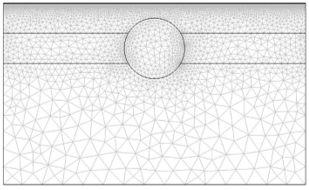Figure 3. Two-dimensional finite element mesh of human skin with embedded tumor model

## 4.png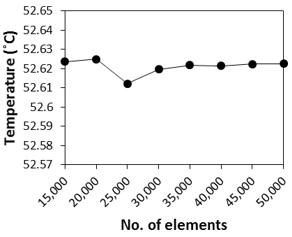Figure 4. Grid convergence curve of the model

4. Results and Discussion

In this study, the effects of wavelength, laser intensity, laser beam area, tumor absorption coefficient, tumor position, irradiated duration time and tumor blood perfusion rate on the temperature distributions in the skin embedded tumor during laser-induced thermotherapy are systematically investigated. The following discussion focuses on the heat transfer that occurs within four layered skin with an embedded tumor during laser-induced thermotherapy.

4.1 Verification of the model

## 5.png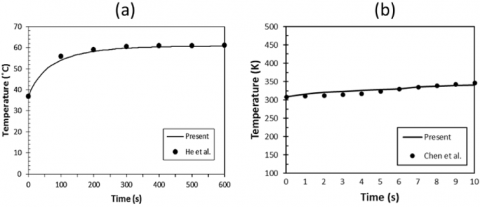Figure 5. Comparison of temperature distribution values between the calculated present result and result obtained by (a) He et al., 2004  and (b) Chen et al., 2014 

To verify the accuracy of the present numerical model, the modified cases of the simulated results are then validated against the numerical results with the same geometric model obtained by He et al.  and Chen et al.  as shown in Figure 5(a)-(b). The axially symmetric two layered human skin tissue with embedded tumor was used in He et al. . The laser irradiation is exposed to the skin with a laser irradiation intensity of 1.4 W. mm-2. Then, the results of the selected test case of He et al.  are illustrated in Figure 5(a) for the temperature distribution in human skin tissue with embedded tumor.

Figure 5(b) represents mathematical simulation results obtained by Chen et al., 2014 . The model is consisted of 3 layers, epidermis, dermis and subcutaneous fat, and it is simulated with laser wavelength of 532 nm and laser intensity is set at 2 W. mm-2. The result of temperature with elapsed time which located at the center point of laser beam, are demonstrated. The comparison of results between Chen et al., 2014’s  and present result are made. A good agreement between the present solution and both of He et al., 2004  and Chen et al., 2014  are obviously indicated. This favorable comparison lends confidence in the accuracy of the present numerical model. It is important to note that some errors might be occur in the simulations that are generated by the input thermal properties, optical properties and numerical scheme.

4.2 Temperature distribution

The effects of laser wavelength, intensity, beam area, tumor absorption coefficient, tumor position, tumor blood perfusion rate and duration time of irradiation on the temperature distributions in tissue during laser-induced thermotherapy are systematically carried out as shown in Figures 6-16.

## 6.png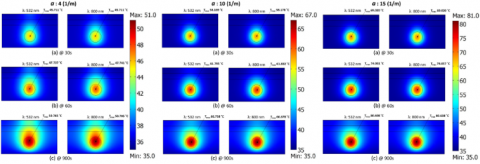Figure 6. Temperature distribution in skin embedded tumor after laser irradiation at 532 and 800 nm wavelengths; the laser irradiation intensity of 1 W. mm-2, the laser beam radius of 1 mm and the tumor absorption coefficients of 4 m-1, 10 m-1 and 15 m-1. (a)-(c) correspond to irradiation durations of 30 s, 60 s and 900 s, respectively

## 7.pngFigure 7. Temperature distribution in skin embedded tumor after laser irradiation at 800 nm wavelengths; laser irradiation intensities of 1 W. mm-2 and 2 W. mm-2, laser beam radius of 1 mm and the tumor absorption coefficients of 4 m-1, 10 m-1 and 15 m-1. (a)-(c) correspond to irradiation durations of 30 s, 60 s and 900 s, respectively

## 8.pngFigure 8. Temperature distribution in skin embedded tumor with different tumor positions after laser irradiation (at irradiation time 900 s); the wavelength of 532 nm, the laser beam radius of 1 mm and the tumor absorption coefficients of 4 m-1, 10 m-1 and 15 m-1. (a)-(b) correspond to laser irradiation intensities of 1 W. mm-2 and 2 W. mm-2, respectively

During procedure of laser-induced thermotherapy, it is necessary to control thermal energy within tissue in order to avoid undesired thermal damage that may exceed appropriate temperature value, or may spread to adjacent healthy tissue around tumor. The temperature increase during laser-induced thermotherapy mainly depends on irradiation time, laser irradiation intensity, laser beam area, tumor absorption coefficient, tumor position, tumor blood perfusion rate and the type of tissues exposed to the laser beam. The following discussion will refer to all of these parameters.

Figure 6 and Figure 7 show the temperature fields in skin tissues after the laser-induced thermotherapy process with three different tumor absorption coefficients (4 m-1, 10 m-1 and 15 m-1) with two wavelengths in Fig 6 (532 nm and 800 nm) and two intensities in Figure 7 (1 W. mm-2 and 2 W. mm-2), at irradiation durations of 30 s, 60 s and 900 s and an irradiated area width of 2 mm. Overall, this simulated results show that the temperature increases with increasing time as well as intensity and tumor absorption coefficient, and temperature reach the highest point at inside the tumor for all cases.

In Figure 6, the effect of wavelength is studied. It found that the maximum temperatures that occurred at 532 nm and 800 nm with different tumor absorption coefficients at time 30 s and 60 s were not constant. However, when the time increased to 900 s, it can be seen that the highest temperature at 532 nm is a little bit higher than at 800 nm because of the optical properties.

## 9.png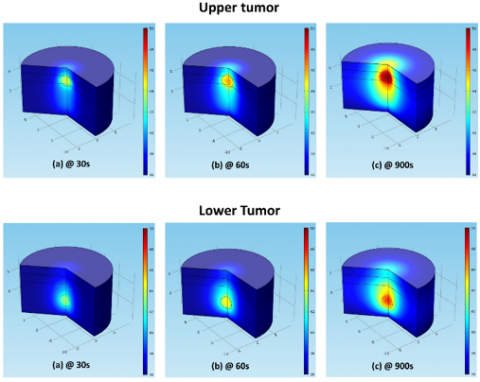Figure 9. 3D temperature distribution in skin embedded tumor with different tumor positions after laser irradiation; the wavelength of 532 nm, the laser beam radius of 2 mm, the tumor absorption coefficients of 4 m-1 and laser irradiation intensity of 1 W. mm-2. (a)-(b) correspond to irradiation times of 30 s, 60 s and 900 s, respectively

## 10.png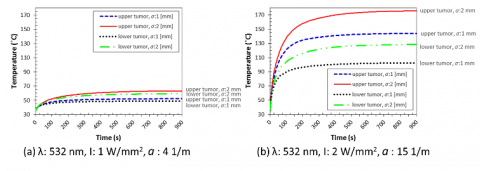Figure 10. Comparison of temperature changes with elapsed times of different tumor positions (upper and lower tumor) and laser beam radius (1 mm and 2 mm) at the highest point of the tumor surface; the wavelength of 532 nm. (a)-(b) Temperature change of laser irradiation intensities 1 W. mm-2 and 2 W. mm-2 with tumor absorption coefficients of 4 m-1 and 15 m-1, respectively

## 11.png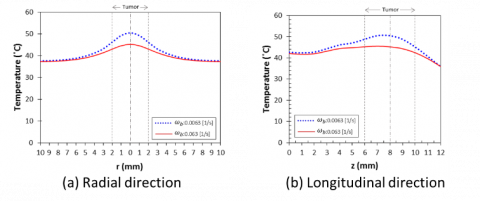Figure 11. Temperature distribution of skin embedded tumor along the radial and longitudinal direction of wavelength 532 nm; tumor blood perfusion rates of 0.0063 s-1 and 0.063 s-1 at an irradiation time of 900 s, the laser irradiation intensity of 1 W. mm-2, tumor absorption coefficient of 4 m-1 and laser beam radius of 1 mm. (a)-(b) correspond to the radial direction and longitudinal direction, respectively

## 12.png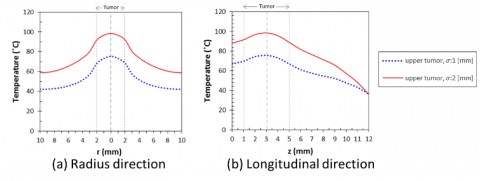Figure 12. Comparison of temperature changes of different laser beam radius (1 mm and 2 mm) along the radial direction and longitudinal direction at the highest point of the tumor surface; the wavelength of 532 nm, laser irradiation intensity of 2 W. mm-2 and tumor absorption coefficient of 4 m-1. (a)-(b) Temperature change of the radial direction and longitudinal direction, respectively

When considering the laser irradiation intensity, the graphs clearly demonstrate the same result as in Figure 7 and Figure 8 that when more intensity is applied, it will lead to increased temperature in both of directions and both tumor positions because the increasing laser irradiation intensity level provides more energy absorption, and then energy is converted to thermal energy as well as increased temperature. In addition, it has more temperature differences inside the tumor when more intensity is applied in both tumor positions because of its difference in optical properties. When deliberate about the position of the tumor, at the same value of intensity, the irradiation time and tumor absorption coefficient, the upper tumor has a higher temperature than the lower tumor in both the radial and longitudinal directions. The effect of tumor position is also obviously indicated in Figure 8, the temperature distribution with different tumor positions at 900s are shown. The result demonstrates that the upper tumor has a higher temperature than the lower tumor in all cases. Furthermore, the results show that when temperature at the embedded tumor (upper and lower positions) has been found to be several degrees higher than that of healthy tissue at the surrounding area when the tumor absorption coefficient is increased.

In this study, a wavelength of 532 nm, laser irradiation intensity of 1 W. mm-2, tumor absorption coefficient of 4 m-1 and irradiation beam area of 2 mm served as an affiant enhancing thermal response in laser-induced thermotherapy throughout the treatment process without side effects to healthy tissue in the surrounding area. Therefore, the simulated temperature changes in a skin embedded tumor, especially the hot spot zone in an embedded tumor with elapsed times are depicted in a three-dimensional plane, as shown in Figure 9. In the figures, it is clearly observed that the embedded tumor has sensitivity to the thermal response, such that the maximum temperature increase over the irradiation time at a point on the boundary of the embedded tumor could not exceed more than 60℃.

Figure 10 shows the irradiated area coupled with tumor position. Figure 10 demonstrates the comparison of temperature changes with the elapsed time of the lowest temperature case, Figure 10(a) (intensity 1 W. mm-2, absorption coefficient 4 m-1) and the highest temperature case, Figure 11(b) (intensity 2 W. mm-2, absorption coefficient 15 m-1) for different tumor positions (upper and lower tumor) coupled with irradiated areas (2 mm and 4 mm) at the highest point of the tumor surface. In the lowest temperature case in Figure 10(a), the irradiated area has more influence than tumor position. The highest temperature value is on 4 mm irradiated area in both tumor positions. The highest temperature case in Figure 10(b) is different. The result shows that the upper tumor has the highest temperature value for both irradiated area widths. Thus, it can be inferred that increasing the intensity and tumor absorption coefficient enhance the effect of the tumor position.

It has long been revealed that existence of a malignant embedded tumor often leads to different blood perfusion rates in the tumor and the surrounding tissue . Therefore, the discussion on the effect of tumor blood perfusion rate is carried out in Figure 11. Figure 11 (a)-(b) demonstrate the temperature change in the skin embedded tumor along the radial and longitudinal directions of wavelength 532 nm, where the tumor blood perfusion rates are 0.0063 s-1 and 0.063 s-1 at an irradiation time of 900 s, respectively; the laser irradiation intensity is 1 W. mm-2, tumor absorption coefficient is 4 m-1 and irradiated area width is 2 mm. It found that, tumor blood perfusion rate has an effect on temperature change within the tumor. When blood perfusion rate of tumor is increasing, it shows decreasing in temperature value because of convective heat transfer plays important role. Then the relationship between blood perfusion rate and temperature change is inverse. This result confirms the significant increased blood perfusion rate as one of the indicators of comer that is recognized widely in the medical literature.

## 13.png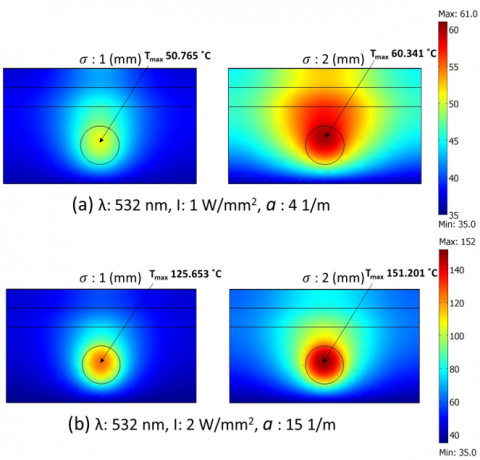Figure 13. Temperature distribution in skin embedded tumor after laser irradiation of different laser beam radius, 1 mm and 2 mm; the wavelength of 532 nm at an irradiation time of 900 s. (a) Temperature distribution of laser irradiation intensity 1 W. mm-2 and tumor absorption coefficients 4 m-1. (b) Temperature distribution of laser irradiation intensity 2 W. mm-2 and tumor absorption coefficients 15 m-1

The clinical safety of skin cancer treatment should be cogitated. Hyperthermia is one of technique that can applied for cancer treatment procedures. This technique, the targeted tissue, as well as the tumor, must be evaluated at temperatures in the specified range of 46-50℃ to cause cancerous cell or tumor death resulting from the enzymatic processes. This range of temperature level is accepted as being optimal for cancer treatment procedures or other applications for hyperthermia treatment.

Increasing some parameters can lead to more energy absorption and cause excess temperature that can destroy the surrounding healthy tissue. The temperature on the tumor surface from this study is shown in Figure 10 and Figure 12. We chose the highest point of the tumor surface because this point is directly impacted from the irradiation direction and has the highest temperature value when compared with other points around the tumor. In Figure 10(a), the temperature elapse time on the tumor surface is in the safety level for all cases. In Figure 10(b), the temperature value is safe only at the early stage (less than 25 s), but after that, the excess temperature value may lead to hyperthermal phenomena that can destroy the surrounding healthy tissue. Moreover, Figure 12 shows the temperature at the tumor age along the radial direction (at r=2 mm) and the longitudinal direction (at z=1 mm and 5 mm). It can imply that the smaller irradiated area (2 mm) is suitable for use in skin cancer treatment than that in a larger irradiated area (4 mm). This is because the effect of thermal conductivities plays an important role in the conductance of the laser energy that is absorbed. To summarize, the irradiation time and the irradiated area should be small enough to protect healthy tissue that is destroyed from heat diffusion.

The effect of the irradiation beam area is investigated in Figure 12 and Figure 13. Figure 12 shows the comparison of the temperature changes with elapsed time of different irradiation beam areas (2 mm and 4 mm) along the radial (Figure 12(a)) and longitudinal directions (Figure 12(b)); at the highest point of the tumor surface, the wavelength is 532 nm, the laser irradiation intensity is 2 W. mm-2 and absorption coefficient is 4 m-1. Figure 13 indicates the temperature distribution in the skin embedded tumor after laser irradiation of different beam areas (diameter 2 mm and 4 mm) in the lowest temperature case, Figure 13(a) (intensity 1 W. mm-2, absorption coefficient 4 m-1) and the highest temperature case, Figure 13(b) (intensity 2 W. mm-2, absorption coefficient 15 m-1) with the same wavelength (532 nm) and an irradiation time of 900 s. The result shows that the greater beam area (4 mm) gives a higher maximum temperature than a smaller area (2 mm) in both the lower and higher temperature cases because of the effect of thermal and optical properties. It can be seen that the result of Figure 12 is similar with Figure 13, the greater beam area provides a greater temperature in both directions inside the tumor because of its thermal and optical properties.

The above results confirm that the increasing irradiation beam area would strongly enhance the sensitivity of the thermal response in the embedded tumor. This is because the larger part of the laser beam is deposited in the targeted tissue. However, for the current case, the beam area of 2 mm seems a safe choice for healthy tissue in the human body.

The temperature distribution of the skin embedded tumor of different tumor absorption coefficients (4 m-1, 10 m-1 and 15 m-1) and different laser radiation intensities (1 W. mm-2 and 2 W. mm-2) along the radial direction and longitudinal direction are shown in Figure 14 and Figure 15, respectively; when the laser duration is 900 s, the wavelength is 532 nm and the irradiated area width of 2 mm. Figure 14(a) and Figure 15(a) indicate the temperature change of the upper tumor and Figure 14(b) and Figure 15(b) indicate the temperature change of the lower tumor. To obtain a large temperature increase in the tumor while limiting the normal tissue temperature, it is necessary to increase the local absorptivity of the tumor by injecting the substance that is particularly sensitive to light into the tumor, as mentioned in section 2.

## 14.png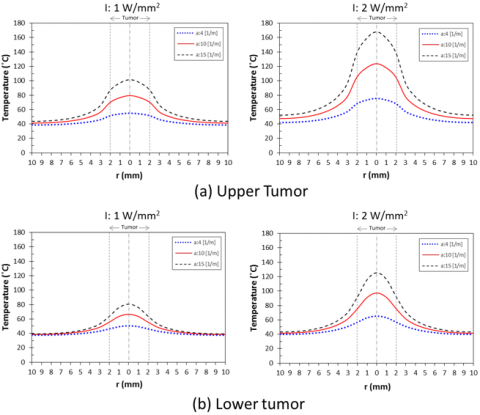Figure 14. Temperature distribution of skin embedded tumor along the radial direction of different tumor absorption coefficients; the time duration of 900 s, the wavelength of 532 nm, laser beam radius of 1 mm, tumor absorption coefficients of 4 m-1, 10 m-1 and 15 m-1 and laser irradiation intensities of 1 W. mm-2 and 2 W. mm-2, respectively. (a)-(b) correspond to the temperature distribution along the radial direction on the tumor center of the upper and lower tumors, respectively

## 15.png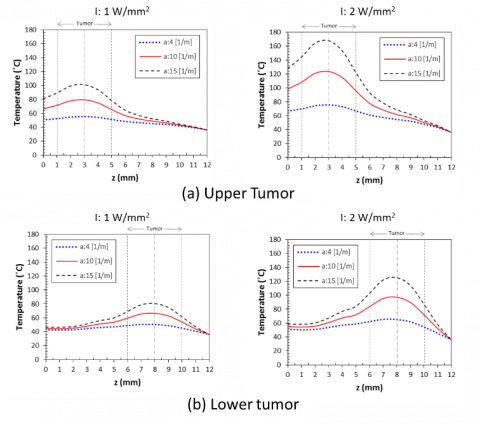Figure 15. Temperature distribution of skin embedded tumor along the longitudinal direction of different tumor absorption coefficients; the time duration of 900 s, the wavelength of 532 nm, laser beam radius of 1 mm, tumor absorption coefficients of 4 m-1, 10 m-1 and 15 m-1 and laser irradiation intensities of 1 W. mm-2 and 2 W. mm-2, respectively. (a)-(b) correspond to the temperature distribution along the longitudinal direction on the tumor center of the upper and lower tumors, respectively

## 16.png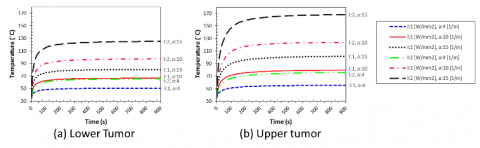Figure 16. Temperature changes (at the tumor center) with elapsed times at different laser irradiation intensities (1 W. mm-2 and 2 W. mm-2) of 532 nm wavelength; the tumor absorption coefficients of 4 m-1, 10 m-1 and 15 m-1 and the laser beam radius of 1 mm. (a)-(b) temperature changes of the lower tumor and upper tumor, respectively

The effect of tumor absorption coefficients is also investigated. Practically, in light of laser photon enhanced absorption, better sensitivity of the thermal response in embedded tumors has been obtained by injecting some substances to the targeted tumor. As depicted in Figure 14 and Figure 15, increasing the tumor absorption coefficient from 4 to 15 m-1, the maximum temperature in the embedded tumor will increase with increasing tumor absorption coefficient for the upper and lower tumor positions, because of its optical properties, and that are related to the result in Figure 6, Figure 7 and Figure 8. Therefore, by means of increasing the tumor absorption coefficient or in another words, increasing concentration of nanoparticles, it can enhance the absorption of the laser in the targeted tissue. However, for safety, the selected suitable value of the tumor absorption coefficient is still considered to be the best choice for enhancing the main thermal fermentations of the tumor. In this study, for good performance, the tumor absorption coefficient of 4 m-1 can serve as an affiant enhancing thermal response in laser-induced thermotherapy throughout the treatment process without side effects to healthy tissue in the surrounding area.

The temperature changes at the tumor center with elapsed times at different laser irradiation intensities (1 W. mm-2 and 2 W. mm-2) and different tumor absorption coefficients (4 m-1, 10 m-1 and 15 m-1) are shown in Figure 16. The effect of the laser irradiation intensity coupled with the tumor absorption coefficient is analyzed. The figures show both tumor positions; intensities of 2 W. mm-2 coupled with absorption coefficients 15 m-1 have the highest temperature. The second highest temperature is intensities of 2 W. mm-2 coupled with absorption coefficients of 10 m-1. The third and fourth highest temperatures occurred at intensities of 1 W. mm-2 coupled with absorption coefficients of 15 m-1 and intensities of 1 W. mm-2 coupled with absorption coefficients of 10 m-1; the tumor absorption coefficient has a more significant influence than laser irradiation intensity.

There are two steps during laser-induced thermotherapy. Namely, the tissue is first heated directly within the optical absorption depth and is followed by heat diffusion to the surrounding tissue. Generally, at the early stage of laser-induced thermotherapy, the heat diffusion does not reach wider into the surrounding tissues. Then, heat diffusion has sufficient time to spread into tissues. The differences of temperature fields in skin tissues in each testing condition are a result of the effect of optical properties, i.e., the absorption coefficient of the epidermis layer, and its value varies with wavelength; the effect of thermal parameters of tissues in each layer are much different. In addition, at the early stage, the blood perfusion rate resulting from convection prevents the temperature from increasing even more.

5. Conclusion

The numerical simulation of heat transfer in human skin during laser ablation therapy in various conditions is represented in this study. Parametric studies on thermal enhanced effects for laser-induced thermotherapy in multilayered skin (epidermis, dermis, subcutaneous fat and muscle) with an embedded tumor such as by varying wavelength, laser intensity, beam area, tumor absorption coefficient, tumor position tumor blood perfusion rate and irradiation times disclose a more quantitative mechanism for achieving the optimum condition for cancerous tumor treatment by laser.

The results show that, the temperature results within tumor are significantly different for different parameters such as laser irradiation intensity, tumor absorption coefficient, laser beam area, tumor position and tumor blood perfusion rate. It found that the greater irradiated intensity and tumor absorption coefficient result in greater heat generation inside skin tissue, thereby increasing the rate of temperature increase. It also found that the temperature change within human skin induced by laser irradiation are not directly related to intensity, the effects of the tumor absorption coefficient, tumor position, irradiated area, thermal and optical properties and blood perfusion rate are also have influence to thermal phenomena within skin tissue.

The effectiveness of treatment result is depended on how appropriate of specified values are set to suit with tumor location (tumor depth), size and concentration of energy adsorbent substance which is injected into target tumor (absorption coefficient). These values such as wavelength, intensity, beam area as well as irradiation time, all affect to temperature distribution and penetration depth. To describe in depth, tumor which is located at shallow skin can absorb more energy when compare with deeper one, due to it is located closer to power generator. Therefore, wavelength, intensity and related parameter should be carefully chosen.

The obtained values provide an indication of limitations that must be considered for laser ablation. Moreover, the obtained results represent the phenomena accurately to determine the temperature increase in the tumor within the skin and make it possible to recommend a guideline to indicate appropriate laser power, irradiation time and related parameters that must be considered during laser ablation treatment.

From the clinical safety point of view for skin cancer treatment, according to the simulated results, the results indicate the following facts: (1) The parameters could be selected to significantly threaten the embedded tumor with thermal response sensitivity, such that the maximum temperature increase over the irradiation time at a point on the boundary of the embedded tumor could not be more than 60˚C. (2) Regarding the safety concern for healthy tissues, the default parameter setting, namely, a laser irradiation intensity of 1 W. mm-2, tumor absorption coefficient of 4 m-1 and irradiation beam area of 2 mm, can be a good choice in overall cancer treatment procedures. (3) The higher tumor absorption coefficient value, by injecting the nanoparticles substance that accumulated in the embedded tumor and increasing the local absorptivity in the embedded tumor (10 or 15 m-1), can be considered as an extremely useful substance for enhancing the thermal response, especially in the very short period of the laser-induced thermotherapy process. They also can be effectively applied in cases with deeper positions of embedded tumors.

For future work, a more complex tissue layer in three dimensions or even in these dimensional spaces for the domains corresponding to the embedded tumor and the surrounding healthy tissue will be carried out. Moreover, the skin bio-thermomechanics will be modeled in future work.

Acknowledgements

The authors gratefully acknowledge the Thailand Science Research and Innovation (Contract No. MRG 6280069), and the Program Management Unit for Human Resources & Institutional Development, Research and Innovation, NXPO (grant number B05F630092) provided financial support for this study.

Nomenclature
 a absorption coefficient, m-1 b scattering coefficient, m-1 C specific heat, J .kg-1. K-1 h convection heat transfer coefficient, W. m-2. K-1 I laser irradiation intensity, W. mm-2 k thermal conductivity, W. m-1. K-1 Q heat generation, W. m-3 r width of tissue, mm T temperature, ℃ z depth of tissue, mm Greek symbols $\rho$ density, kg. m-3 ω perfusion, 1. s-1 σ The radius of laser beam, mm Subscripts 0 initial, skin surface am ambient b blood cb core body met metabolic
References

 Narayanan, D.L., Saladi, R.N., Fox, J.L. (2010). Ultraviolet radiation and skin cancer. International Journal of Dermatology, 49(9): 978-986. https://doi.org/10.1111/j.1365-4632.2010.04474.x

 Kumar, V., Abbas, A., Fausto, N., Aster, J. (2009). Robbins and Cotran Pathologic Basis of Disease, Professional Edition, 8th, Elsevier.

 Schena, E., Saccomandi, P., Fong, Y. (2017). Laser ablation for cancer: past, present and future. Journal of Functional Biomaterials, 8(2): 19. https://doi.org/10.3390/jfb8020019

 Pennes, H.H. (1998). Analysis of tissue and arterial blood temperatures in the resting human forearm. Journal of Applied Physiology, 85(1): 5-34. https://doi.org/10.1152/jappl.1998.85.1.5

 Sazgarnia, A., Naghavi, N., Mehdizadeh, H., Shahamat, Z. (2015). Investigation of thermal distribution for pulsed laser radiation in cancer treatment with nanoparticle-mediated hyperthermia. Journal of Thermal Biology, 47: 32-41. https://doi.org/10.1016/j.jtherbio.2014.10.011

 Bhowmik, A., Repaka, R., Mishra, S.C., Mitra, K. (2016). Thermal assessment of ablation limit of subsurface tumor during focused ultrasound and laser heating. Journal of Thermal Science and Engineering Applications, 8(1): 011012. https://doi.org/10.1115/1.4030731

 Luna, J.M., Hernández Guerrero, A., Romero Méndez, R., Luviano Ortiz, J.L. (2014). Solution of the inverse bio-heat transfer problem for a simplified dermatological application: case of skin cancer. Ingeniería Mecánica, Tecnología y Desarrollo, 4(6): 219-228.

 Manuchehrabadi, N., Chen, Y., LeBrun, A., Ma, R., Zhu, L. (2013). Computational simulation of temperature elevations in tumors using Monte Carlo method and comparison to experimental measurements in laser photothermal therapy. Journal of Biomechanical Engineering, 135(12). https://doi.org/10.1115/1.4025388

 Fasano, A., Hömberg, D., Naumov, D. (2010). On a mathematical model for laser-induced thermotherapy. Applied Mathematical Modelling, 34(12): 3831-3840. https://doi.org/10.1016/j.apm.2010.03.023

 Marqa, M.F., Colin, P., Nevoux, P., Mordon, S.R., Betrouni, N. (2011). Focal laser ablation of prostate cancer: numerical simulation of temperature and damage distribution. Biomedical Engineering Online, 10(1): 1-12. https://doi.org/10.1186/1475-925X-10-45

 Ma, N., Gao, X., Zhang, X.X. (2004). Two-layer simulation model of laser-induced interstitial thermo-therapy. Lasers in Medical Science, 18(4): 184-189. https://doi.org/10.1007/s10103-003-0278-2

 Deng, Z.S., Liu, J. (2004). Mathematical modeling of temperature mapping over skin surface and its implementation in thermal disease diagnostics. Computers in Biology and Medicine, 34(6): 495-521. https://doi.org/10.1016/S0010-4825(03)00086-6

 Strong, F.C. (1952). Theoretical basis of Bouguer-Beer law of radiation absorption. Analytical Chemistry, 24(2): 338-342. https://doi.org/10.1021/ac60062a020

 Paul, A., Narasimhan, A., Kahlen, F.J., Das, S.K. (2014). Temperature evolution in tissues embedded with large blood vessels during photo-thermal heating. Journal of Thermal Biology, 41: 77-87. https://doi.org/10.1016/j.jtherbio.2014.02.010

 Fanjul-Vélez, F., Romanov, O.G., Arce-Diego, J.L. (2009). Efficient 3D numerical approach for temperature prediction in laser irradiated biological tissues. Computers in Biology and Medicine, 39(9): 810-817. https://doi.org/10.1016/j.compbiomed.2009.06.009

 Cheng, T.Y., Herman, C. (2014). Analysis of skin cooling for quantitative dynamic infrared imaging of near-surface lesions. International Journal of Thermal Sciences, 86: 175-188. https://doi.org/10.1016/j.ijthermalsci.2014.06.033

 Tseng, S.H., Bargo, P., Durkin, A., Kollias, N. (2009). Chromophore concentrations, absorption and scattering properties of human skin in-vivo. Optics Express, 17(17): 14599-14617. https://doi.org/10.1364/OE.17.014599

 Aguilar, G., Díaz, S.H., Lavernia, E.J., Nelson, J.S. (2002). Cryogen spray cooling efficiency: Improvement of port wine stain laser therapy through multiple‐intermittent cryogen spurts and laser pulses. Lasers in Surgery and Medicine: The Official Journal of the American Society for Laser Medicine and Surgery, 31(1): 27-35. https://doi.org/10.1002/lsm.10076

 He, Y., Minoru, S., Ryu, K., Himeno, R., Kawamura, T. (2004). Numerical and experimental study on the human blood circulation and heat transport phenomena-thermoregulation in the periphery and hyperthermia-induced tumor blood flow, computational biomechanics. RIKEN Symposium, 97-119.

 Jin, C., He, Z.Z., Zhang, J., Yang, X.Y., Liu, J. (2013). Enhanced thermographic detection of skin cancer through combining laser scanning and biodegradable nanoparticles. Journal of Nanotechnology in Engineering and Medicine, 4(1): 011004-011008. https://doi.org/10.1115/1.4024129

 Chen, K., Liang, Y., Zhu, W., Sun, X., Wang, T. (2014). Simulation of temperature distribution in skin under laser irradiation with different wavelengths. Optik, 125(5): 1676-1679. https://doi.org/10.1016/j.ijleo.2013.10.021

 Song, C.W. (1980). Physiological factors in hyperthermia. National Cancer Institute Monograph, 61: 169-176.